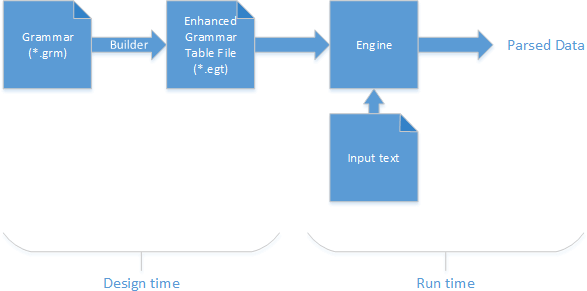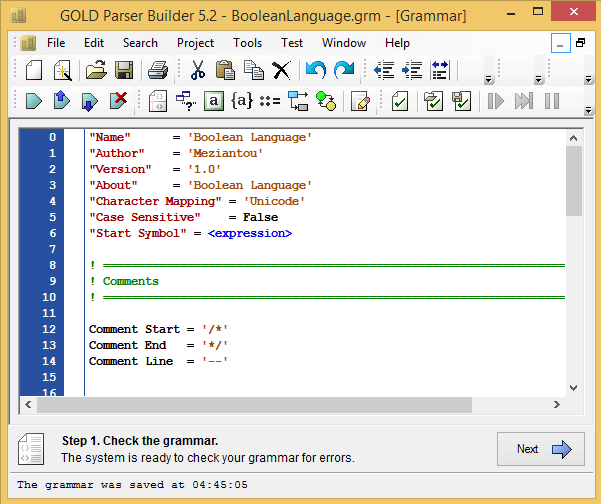# Introduction to GoldParser

In a previous article, I detailed my answer to a question from StackOverflow for which I wrote a Boolean expression parser. At the end of the article I indicated that it was better to generate the parsers with tools such as ANLR or GoldParser. In this article we will see how to use it.

GOLD is a free parsing system that you can use to develop your own programming languages, scripting languages and interpreters. It strives to be a development tool that can be used with numerous programming languages and on multiple platforms.

The way Gold works is as follows:

1. Writing grammar
2. Generating Analysis Tables Using the Builder
3. At runtime these tables are read by the chosen engine and we can start analyzing files. There are different engines to support different languages / platforms.## Creating grammar

In order to create and test the grammar I used GOLD Parser Builder.

The grammar begins with a preamble describing it:

``````"Name"      = 'Boolean Language'
"Author"    = 'Meziantou'
"Version"   = '1.0'
"About"     = 'Boolean Language'
"Character Mapping" = 'Unicode'
"Case Sensitive"    = False
"Start Symbol" = <expression>``````

We then set the comment format (if the grammar contains some):

``````Comment Start = '/*'
Comment End   = '*/'
Comment Line  = '--'``````

Then we define the terminal symbols. Terminal symbols are symbols such as integers, real numbers, strings, dates, and so on.

``````{Id Ch Standard} = {Alphanumeric} + [_] + [.]
{Id Ch Extended} = {Printable} + {Letter Extended} - ['['] - [']']
! Examples: Test; [A B]
Identifier = {Id Ch Standard}+ | '['{Id Ch Extended}+']'
Boolean = 'true' | 'false'``````

Finally, we define the rules in the format Backus-Naur Form (BNF):

``````<expression> ::=
<andExpression>
| <orExpression>
| <xorExpression>
| <subExpression>

<andExpression> ::=
<expression> '&&' <subExpression>
| <expression> 'and' <subExpression>

<orExpression>  ::=
<expression> '||' <subExpression>
| <expression> 'or' <subExpression>

<xorExpression> ::=
<expression> '^' <subExpression>
| <expression> 'xor' <subExpression>

<subExpression> ::=
<parentheseExpression>
| <notExpression>
| <value>

<parentheseExpression> ::= '(' <expression> ')'

<notExpression> ::= '!' <subExpression>

<value> ::=
Boolean
| Identifier``````

With the grammar complete, we can now generate the tables. The interface is clear: click on "next" until you are offered to save the generated file.It is then possible to test the grammar directly in the tool (Test menu):## Some code

Before anything else you have to download the engine for .NET: http://goldparser.org/engine/5/net/index.htm

The outline code breaks down into three stages:

1. Instantiate the analysis engine
2. Load analysis tables generated from grammar
3. Read the file to analyze
4. Create an object for each rule of the grammar (Reduction step). For example, for the `<orExpression>` rule, we create an `OrExpression` object that contains the left expression and the right expression. At the end of the analysis (step `Accept`) we can retrieve the last object thus created. This represents a tree corresponding to the analyzed expression. For example, for the text "[Expert] && ([ReadWrite] || [ReadOnly])" the created tree will be:``````// Instantiate the parser and load the grammar file
Parser parser = new Parser();
using (BinaryReader grammar = GetGrammar())
{
parser.LoadTables(grammar);
}

using (TextReader textReader = new StringReader("true || false"))
{
parser.Open(textReader);
parser.TrimReductions = true;
while (true)
{
// The 4 useful ParseMessage are:
//   Reduction: A rule is matched => we can create an object with its content
//   Accept: End of the parsing => we can get the result
//   LexicalError and SyntaxError: The file is not valid
ParseMessage response = parser.Parse();
switch (response)
{
case ParseMessage.TokenRead:
Trace.WriteLine("Token: " + parser.CurrentToken.Parent.Name);
break;

case ParseMessage.Reduction:
parser.CurrentReduction = CreateNewObject(parser.CurrentReduction as Reduction);
break;

case ParseMessage.Accept:
Expression result = parser.CurrentReduction as Expression;
if (result != null)
{
Console.WriteLine(result.DisplayName);
}
return;

case ParseMessage.LexicalError:
Console.WriteLine("Lexical Error. Line {0}, Column {1}. Token {2} was not expected.",
parser.CurrentPosition.Line,
parser.CurrentPosition.Column,
parser.CurrentToken.Data);
return;

case ParseMessage.SyntaxError:
StringBuilder expecting = new StringBuilder();
foreach (Symbol tokenSymbol in parser.ExpectedSymbols)
{
expecting.Append(' ');
expecting.Append(tokenSymbol);
}

Console.WriteLine("Syntax Error. Line {0}, Column {1}. Expecting: {2}.",
parser.CurrentPosition.Line,
parser.CurrentPosition.Column,
expecting);
return;

case ParseMessage.InternalError:
case ParseMessage.NotLoadedError:
case ParseMessage.GroupError:
return;
}
}
}

static object CreateNewObject(Reduction r)
{
string ruleName = r.Parent.Head.Name;
Trace.WriteLine("Reduce: " + ruleName);
if (ruleName == "orExpression")
{
var left = r.GetData(0) as Expression;
var right = r.GetData(2) as Expression;
return new OrExpression(left, right);
}
else if (ruleName == "andExpression")
{
var left = r.GetData(0) as Expression;
var right = r.GetData(2) as Expression;
return new AndExpression(left, right);
}
/// ...
else if (ruleName == "value")
{
var value = r.GetData(0) as string;
if (value != null)
{
value = value.Trim();
bool boolean;
if (bool.TryParse(value, out boolean))
return new BooleanValueExpression(boolean);

return new RoleNameExpression(value);
}
}
return null;
}``````

The full code is available on GitHub: https://github.com/meziantou/GoldParser-Engine